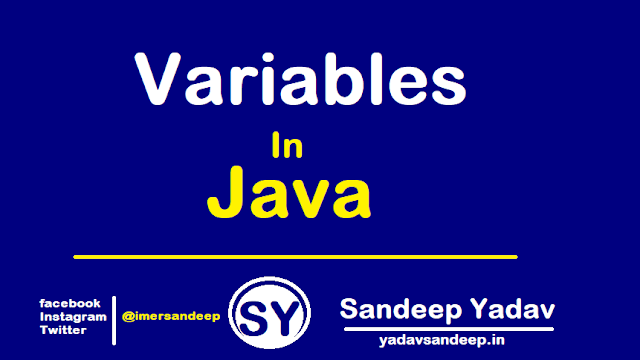# Variables in Java | Types of variable in Java

.
Java variable
A variable is a name which is used to hold the constant value while the java program is executed .
int a=100;
here, variable a is associated with the value 100, and int represent the data type of variable.

How to declare variables ?

Variable declaration in java composed of 4 component
1) Variable type
2) Variable name
3) Zero or more modifier
4) Constant value /Literal

syntax : variable declaration

public int empId =10 ;

Here ,
public : Modifier name
int : Data type
empId :  variable name
10 : Constant value

Types of variable in Java
Variable in java divided into 3 sub parts
1) Local Variable
2) Instance Variable
3) Static Variable

Local Variable

The variable which are defined within a method / blocks / Constructor are know as the local variable .
• Local variable memory allocated when the method starts and destroyed when method completed.
• In java local variable are stored in stack memory .
Scope of local variable : You can access local variable only within that method , blocks , constructor .Out side of the method / blocks  / Constructor not possible .

Example (1): Local Variable

class Test

{
public static void main(String[] args)
{
int a=100;          //Local variable
int b=100;         //Local variable
int c=a+b;         // Local variable
System.out.println(c);
}
}
0utput : 200

Example (2): Local Variable (when try to access out side of the method / block /constructor ).

class Test
{
public static void main(String[] args)
{
int a=100;      //Local variable
int b=100;     //Local variable
System.out.println(a);  //Possible
}
Void add()
{
System.out.println(a+b); // Not Possible
}
}
Output : Compilation error

Instance Variable ( Non-static variable )
The variable which are defined within class but outside of a method  are know as the instance variable .
• Instance variable memory allocated during the time of object creation and destroyed when the object is destroyed.
• In java instance variable are stored in heap memory .
Scope of instance variable : You can access instance variable only within the class .Out side of the class not possible .

Example : Instance variable

class Test

{
int a=100;      //Instance variable
int b=200;     // Instance variable
public static void main(String[] args)
{
Test t = new Test();
System.out.println(t.a);
System.out.println(t.b);
t.add()
}
Void add()
{
System.out.println(a);
System.out.println(b);
}

}
Outpout :
100
200
100
200

Static Variable (class variable )
The variable which are defined within class but outside of a method with the static modifiers are know as the static variable .
• Static variable memory allocated during the time of .class file loading and destroyed when the execution ends.
• In java static variable are stored in method area .
• Static variable can not be local.
Scope of static variable : You can access static variable only within the class .Out side of the class not possible .

Example :  static variable

class Test
{
static int a=100;    //Static variable
static int b=200;   //Static variable
public static void main(String[] args)

{
System.out.println(Test.a);
System.out.println(Test.b);
Test t = new Test();
t.add()

}

Void add()

{

System.out.println(Test.a);
System.out.println(Test.b);

}

}
Outpout :
100
200
100
200

Hope !!! The above lesson of variable in java helpful for you ...

Thank You !
Sandeep Yadav

Tags : Java variables , variables in java , local variables in java , class variable in java , Scope of variables in java , Instance variable in javaVariables in Java